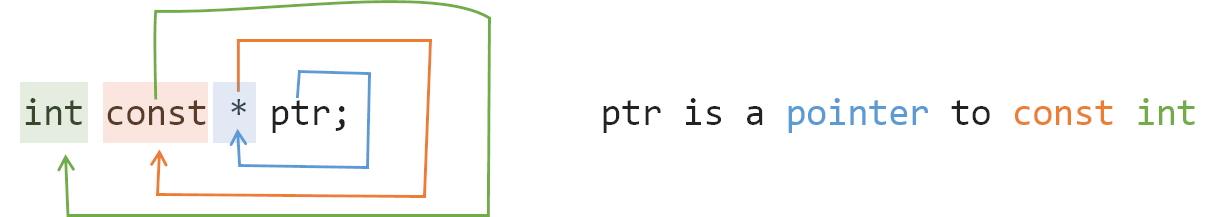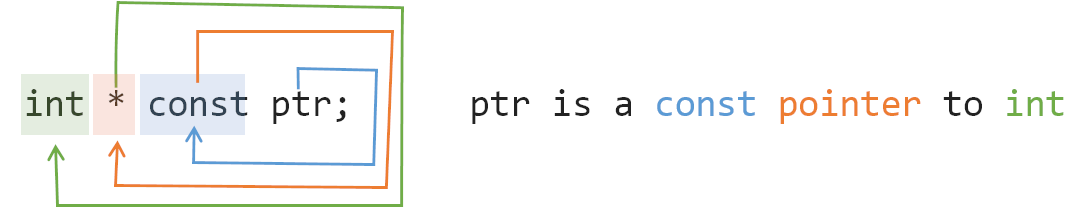# C++ 中让人头晕的const & constexpr

## const

const 一般的用法就是修饰变量、引用、指针，修饰之后它们就变成了常量，需要注意的是 const 并未区分出编译期常量和运行期常量，并且 const 只保证了运行时不直接被修改。

``````const int x = 100; //常量
const int& rx = x; //常量引用
const int* px = &x;//常量指针``````

``````class DemoClass final
{
private:
const long  MAX_SIZE = 256;    // const成员变量
int         m_value;           // 成员变量
public:
int get_value() const        // const成员函数
{
// error: assignment of member ‘DemoClass::m_value’
m_value = 100;
return m_value;
}
};``````

const 用于指针还有其他情况，const 放在声明的最左边，表示指向常量的指针（ pointer to const int），指针指向的是一个“只读变量”，不允许修改：

``````   int x = 100;
const int* px = &x;
*px = 102; // error: assignment of read-only``````

const 放在 “*” 的右边，表示指针是常量（const pointer to int），指针不能被修改，而指向的变量可以被修改：

``````   int x = 100;
int b = 150;
int* const px = &x;
*px = 102; // success
px = &b; // error: assignment of read-only location``````

• `const int *` == `int const *`
• `const int * const` == `int const * const`

``````       +-------+
| +-+   |
| ^ |   |
char *str;
^   ^   |   |
|   +---+   |
+-----------+``````
• 首先想到的是 str 是什么？我们以 str 为顺时针转动，先遇到了 `[` ，所以表示 str 是个数组，所以 str 是一个容量为 10 的数组；
• 然后我们再顺时针移动，下一个遇到的是 `*` ，那么表示这是一个指针，那么 str 是一个容量为 10 的数组指针；
• 我们继续顺时针转动，发现遇到了行结束符 `;`，跳过它继续转动，发现遇到了 `char`，那么表示 str 是一个 char 类型，容量为 10 的数组指针。## constexpr

constexpr 它是在 C++ 11 被引进的，它的字面意思是 constant expression，常量表达式。它可以作用在变量和函数上。一个 constexpr 变量是一个编译时完全确定的常数。一个 constexpr 函数至少对于某一组实参可以在编译期间产生一个编译期常数。

``````template<int N> class C{};

constexpr int FivePlus(int x) {
return 5 + x;
}

void f(const int x) {
C<x> c1;            // Error: x is not compile-time evaluable.
C<FivePlus(6)> c2;  // OK
} ``````

### C++17 & if constexpr

``````template <class T> constexpr T absolute(T arg) {
return arg < 0 ? -arg : arg;
}

template <class T>
constexpr enable_if_t<is_floating_point<T>::value, bool>
close_enough(T a, T b) {
return absolute(a - b) < static_cast<T>(0.000001);
}
template <class T>
constexpr enable_if_t<!is_floating_point<T>::value, bool>
close_enough(T a, T b) {
return a == b;
}``````

``````template <class T> constexpr T absolute(T arg) {
return arg < 0 ? -arg : arg;
}

template <class T>
constexpr auto precision_threshold = T(0.000001);

template <class T> constexpr bool close_enough(T a, T b) {
if constexpr (is_floating_point_v<T>)
return absolute(a - b) < precision_threshold<T>;
else
return a == b;
}``````

## Reference

https://time.geekbang.org/column/article/238486

https://stackoverflow.com/questions/1143262/what-is-the-difference-between-const-int-const-int-const-and-int-const

https://c-faq.com/decl/spiral.anderson.html

https://stackoverflow.com/questions/14116003/whats-the-difference-between-constexpr-and-const

https://www.zhihu.com/question/35614219